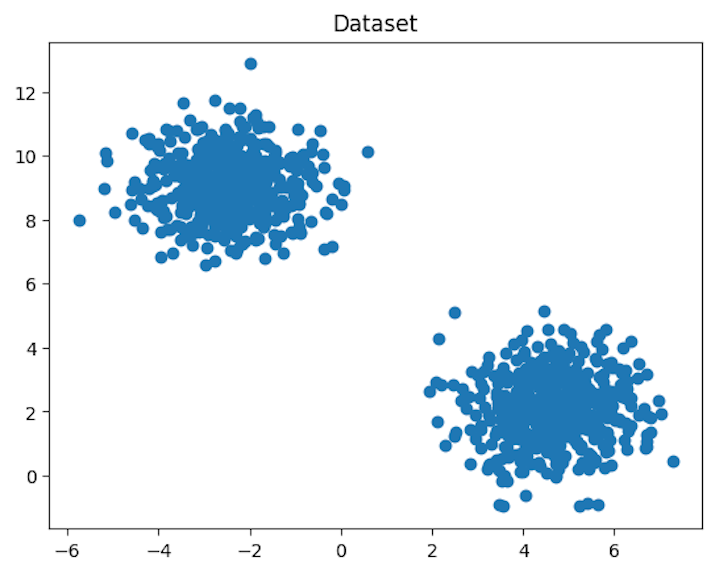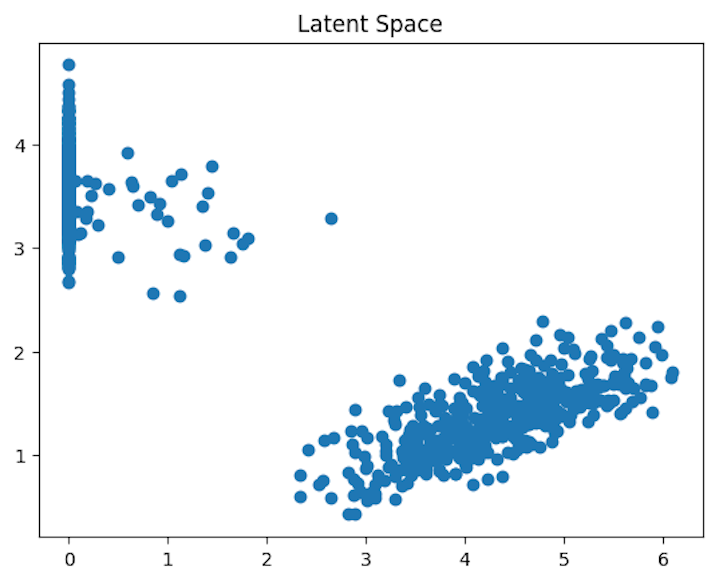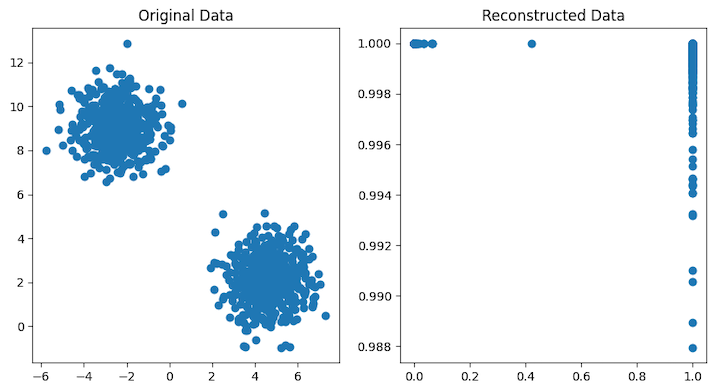# Unsupervised backpropagation in Machine Learning

Artificial intelligence's branch of machine learning gives computers the ability to learn from data and make judgments. A labeled dataset is used to train a model in supervised learning, whereas an unlabeled dataset is used in unsupervised learning. A neural network is used in unsupervised back propagation, a sort of unsupervised learning, to discover patterns in an unlabeled dataset. This blog article will outline unsupervised back propagation before moving on to practical Python code.

## What is unsupervised back propagation?

Back propagation is a supervised learning method that modifies the weights of neural networks to reduce the discrepancy between predicted and observed results. using the other hand, unsupervised back propagation trains the network using unlabeled input to find hidden structures and patterns. In this method, input data is compressed by an auto encoder neural network into a smaller representation known as the latent space, and the original input is then reconstructed using the latent space by a decoder network. Minimizing the reconstruction error between the input data and the output of the decoder network is the aim of unsupervised back propagation.

## Auto encoder Neural Network

The most popular neural network architecture for back propagation unsupervised learning is auto encoders. A neural network called an auto encoder is made up of two neural networks: an encoder network that converts input data into a lower-dimensional representation and a decoder network that converts the lower-dimensional representation back to the original input space.

GANs are a form of machine learning model that consists of two neural networks, one for generation and one for discrimination. While the discriminator tries to tell the difference between actual and bogus data, the generator attempts to produce realistic synthetic data. GANs can be employed in the context of unsupervised back propagation to create fresh data samples devoid of labels. The neural network can then learn from these created samples to find hidden patterns and structures in the data. For problems where labelled data is poor or unavailable, an unsupervised learning strategy utilizing GANs can be useful.

## Unsupervised back propagation using python

To start, we'll build a fictitious dataset with two clusters of data points. With two dense layers in the encoder network and two dense layers in the decoder network, an auto encoder neural network is then built using Keras. Unsupervised back propagation is used to train the auto encoder on the dataset. A scatter plot is used to display the dataset's latent space representation. Using scatter plots, the auto encoder's reconstructed output is lastly shown and contrasted with the data used as input. The outcomes demonstrate that the auto encoder has mastered a suitable model of the dataset.

### Creating dataset

To use in the unsupervised back propagation study, let's develop a particular dataset. Using the scikit-learn tools, a two-dimensional dataset with two clusters of data points will be created.

from sklearn.datasets import make_blobs
X, y = make_blobs(n_samples=1000, centers=2, n_features=2, random_state=42)


A dataset of 1000 data points with two attributes is created using the code displayed above. The centres of the two clusters in the dataset are, respectively, (-5, 0) and (5, 0).

### Visualizing the Dataset

Let's use a scatter plot to visualize the dataset to identify the two data point groupings.

import matplotlib.pyplot as plt

plt.scatter(X[:, 0], X[:, 1])
plt.title('Dataset')
plt.show()


### OutputThe scatter plot reveals that the dataset contains two clusters of data points.

### Defining the Auto encoder Model

Using the Keras package, let's define the auto encoder neural network. A decoder network and an encoder network make up the auto encoder. The decoder network is made up of two dense layers with a sigmoid activation function, while the encoder network is made up of two dense layers with a ReLU activation function.

from keras.layers import Input, Dense
from keras.models import Model

input_layer = Input(shape=(2,))
encoded = Dense(32, activation='relu')(input_layer)
encoded = Dense(16, activation='relu')(encoded)
decoded = Dense(32, activation='relu')(encoded)
decoded = Dense(2, activation='sigmoid')(decoded)
autoencoder = Model(inputs=input_layer, outputs=decoded)


To correspond with the dimensionality of our dataset, we construct an input layer with two dimensions in the code above. Next, we define the encoder network, which consists of two dense layers with ReLU activation functions. Latent space representation is the encoder network's output. Two dense layers with sigmoid activation functions are considered to be the decoder network. The decoder network produces a rebuilt output with the same dimensions as the input.

### Training the Auto encoder Model

After defining the autoencoder neural network, let's use unsupervised back propagation to train it on our dataset. The model will be trained using the fit() technique.

auto encoder.fit(X, X, epochs=50, batch_size=32)


We train the auto encoder model in the code above using the fit() technique. Since the objective is to recreate the input data, we feed in the original data as both the input and the target output. We also define the batch size and the number of epochs to train the model over.

### Visualizing the Latent Space

We can use the encoder network to retrieve the latent space representation of our dataset once the auto encoder has been trained. Afterward, a scatter plot can be used to see the latent space.

encoder = Model(inputs=input_layer, outputs=encoded)
latent_space = encoder.predict(X)

plt.scatter(latent_space[:, 0], latent_space[:, 1])
plt.title('Latent Space')
plt.show()


In the above code, we introduce a brand-new model called an encoder that receives the input layer as input and outputs the latent space representation from the second dense layer of the encoder network. Our dataset's latent space representation is then obtained using the encoder model. A scatter plot is used to depict the latent space.The scatter figure shows that our dataset's latent space representation clearly distinguishes between the two groups of data points, demonstrating that the auto encoder has learned a usable representation of the dataset.

### Visualizing the Reconstructed Output

Let's finally use the input data to run the auto encoder and compare the output to the original input data in order to see how the output was rebuilt.

reconstructed = autoencoder.predict(X)

fig, axes = plt.subplots(1, 2, figsize=(10, 5))
axes.scatter(X[:, 0], X[:, 1])
axes.set_title('Original Data')
axes.scatter(reconstructed[:, 0], reconstructed[:, 1])
axes.set_title('Reconstructed Data')
plt.show()


In the code above, input data is processed via an auto encoder to produce reconstructed output, which is then visualized using scatter plots together with the original data.The scatter plots show that the auto encoder's rebuilt output closely matches the original input data, demonstrating that it has learned a reliable representation of the dataset.

## Conclusion

Finally, unsupervised back propagation is a potent neural network approach for unsupervised learning. Without utilizing labeled training data, it includes teaching a neural network how to represent the incoming data. Clustering, anomaly detection, and dimensionality reduction are just a few unsupervised learning problems that can be handled with this method. As they can acquire usable representations of high-dimensional data without the requirement for labeled training data, auto encoders are a common form of neural network used in unsupervised learning. Machine learning practitioners can apply unsupervised back propagation and auto encoders to their own datasets and glean insightful knowledge from the data by mastering these approaches.

Updated on: 31-Jul-2023

130 Views